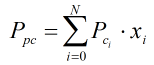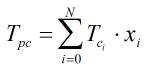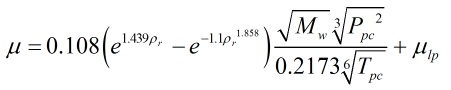# C297 – API Natural Gas Viscosity Calculation

## Description

This calculates the viscosity of a natural gas composition using the procedure outlined in the API Technical Data Book.

API Technical Data Book

Kelton calculation reference C297

FLOCALC calculation reference F094

## Calculation

### Pseudocritical Pressure

The pseudocritical pressure is calculated by:Where Pci = Component critical pressure xi = Component mole fraction

### Pseudocritical Temperature

The pseudocritical temperature is calculated by:Where Tci = Component critical temperature xi = Component mole fraction

### Viscosity

The viscosity of the gas composition is calculated by:Where Mw = Molecular weight ρr = Reduced density μlp = Low pressure viscosity

Back